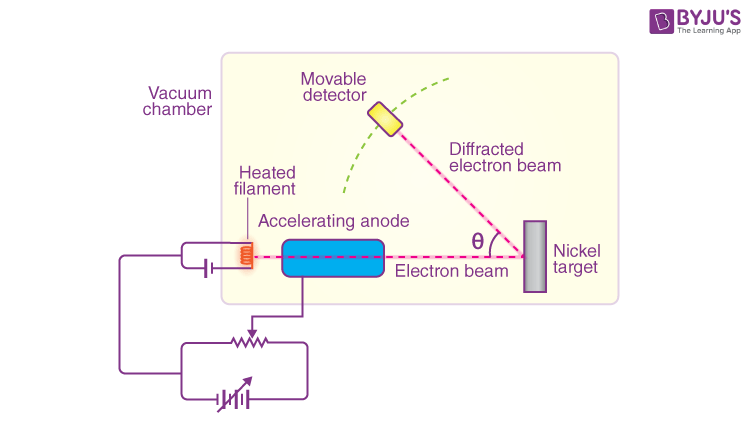# Davisson Germer Experiment - Electron Diffraction

Initial atomic models proposed by scientists could only explain the particle nature of electrons but failed to explain the properties related to their wave nature. C.J. Davisson and L.H. Germer, in the year 1927, carried out an experiment, popularly known as Davisson Germer’s experiment, to explain the wave nature of electrons through electron diffraction. In this article, we will learn about the observations and conclusions of the experiment.

## Setup of Davisson Germer ExperimentThe experimental arrangement of the Davisson Germer experiment is discussed below:

• An electron gun comprising a tungsten filament F was coated with barium oxide and heated through a low voltage power supply.
• While applying suitable potential difference from a high voltage power supply, the electron gun emits electrons which were again accelerated to a particular velocity.
• In a cylinder perforated with fine holes along its axis, these emitted electrons were made to pass through it, thus producing a fine collimated beam.
• The beam produced from the cylinder is again made to fall on the surface of a nickel crystal. Due to this, the electrons scatter in various directions.
• The beam of electrons produced has a certain amount of intensity which is measured by the electron detector and after it is connected to a sensitive galvanometer (to record the current), it is then moved on a circular scale.
• By moving the detector on the circular scale at different positions that is changing the θ (angle between the incident and the scattered electron beams), the intensity of the scattered electron beam is measured for different values of angle of scattering.

Read More: Wave Nature of Matter

### Observations of Davisson Germer experiment:

From this experiment, we can derive the below observations:

• We obtained the variation of the intensity (I) of the scattered electrons by changing the angle of scattering, θ.
• By changing the accelerating potential difference, the accelerated voltage was varied from 44V to 68 V.
• With the intensity (I) of the scattered electron for an accelerating voltage of 54V at a scattering angle θ = 50º, we could see a strong peak in the intensity.
• This peak was the result of constructive interference of the electrons scattered from different layers of the regularly spaced atoms of the crystals.
• With the help of electron diffraction, the wavelength of matter waves was calculated to be 0.165 nm.

### Co-relating Davisson Germer Experiment and de Broglie Relation:

According to de Broglie,

λ = h /p

$$\begin{array}{l}λ = (\frac{1.227}{\sqrt{54}}) = 0.167 nm\end{array}$$

λ = wavelength associated with electrons

Thus, Davisson Germer experiment confirms the wave nature of electrons and the de Broglie relation.